# Presentation on calculus

26. Nov 2014тАвтАв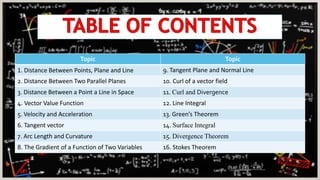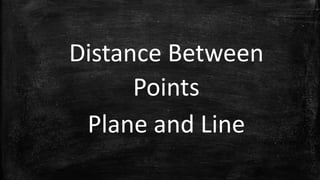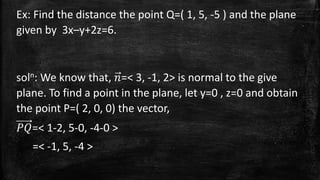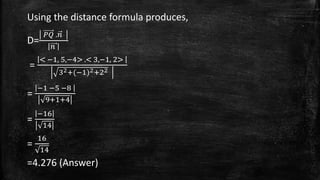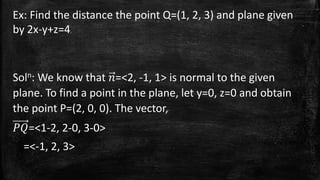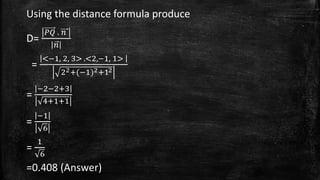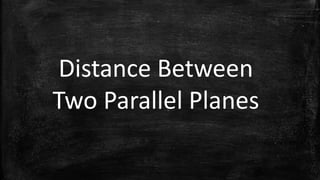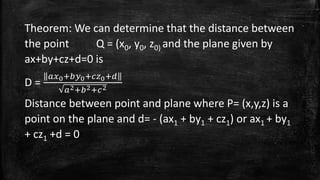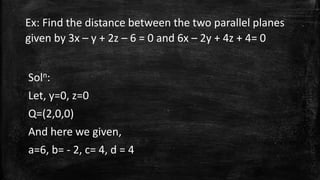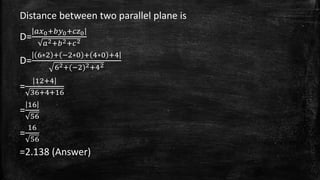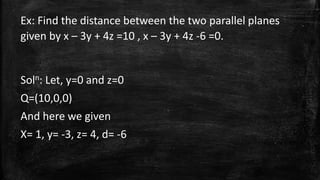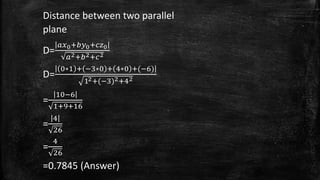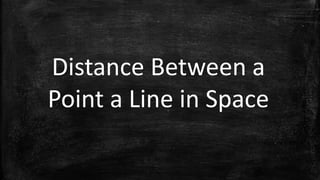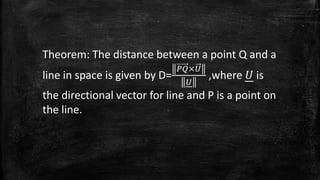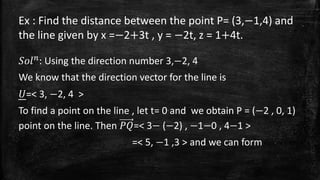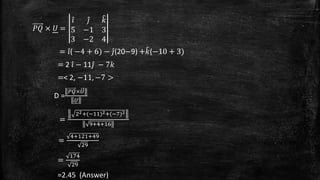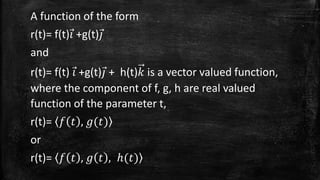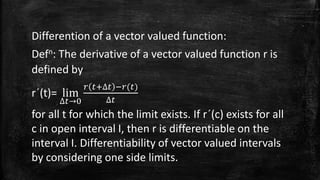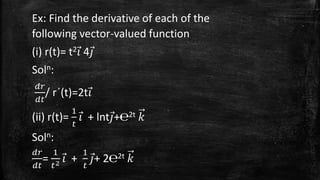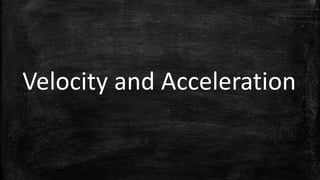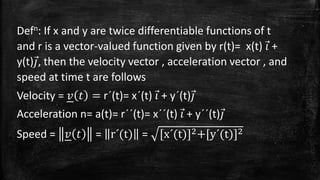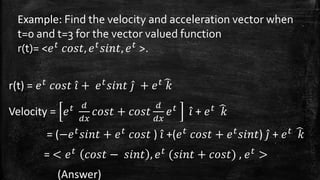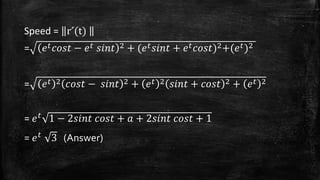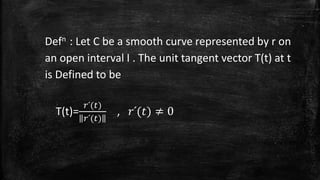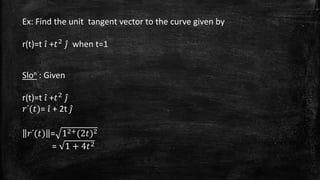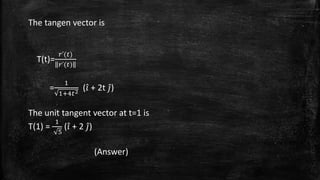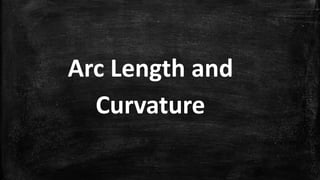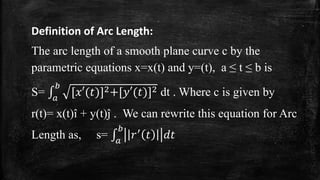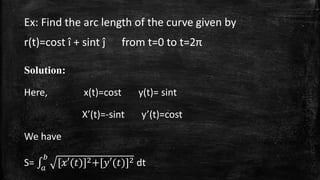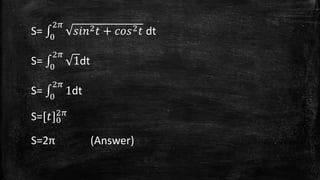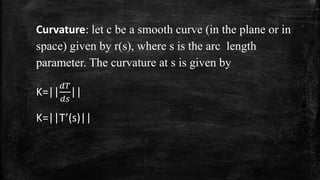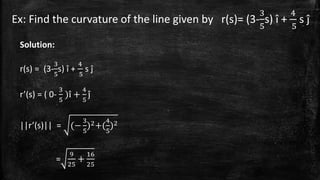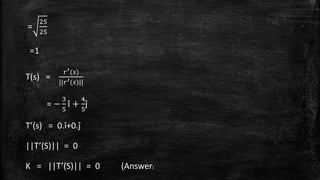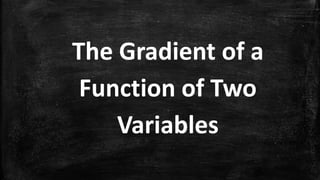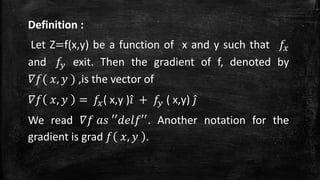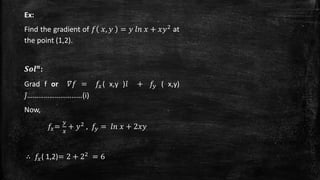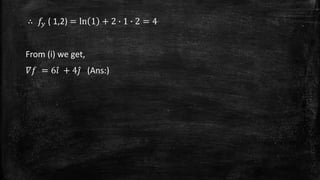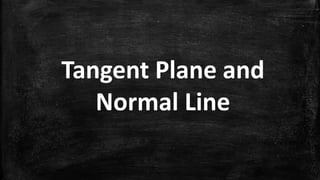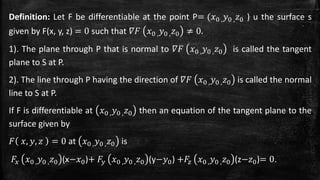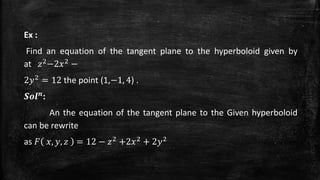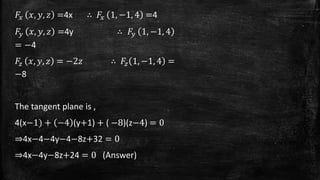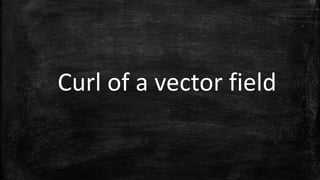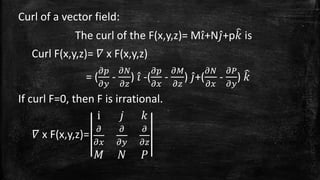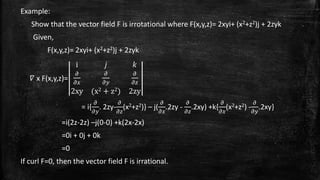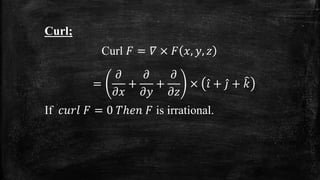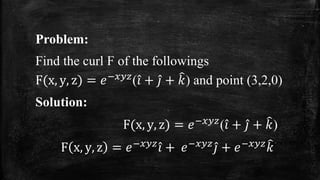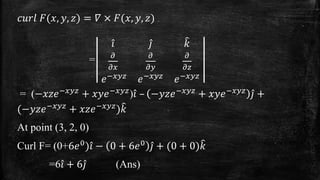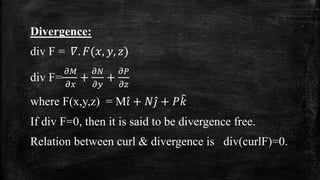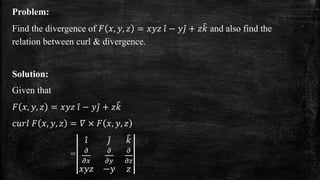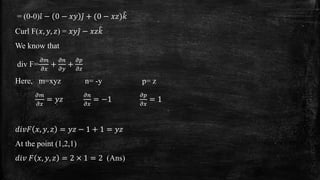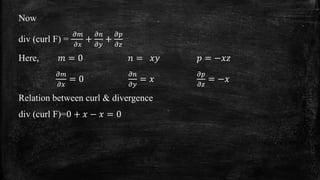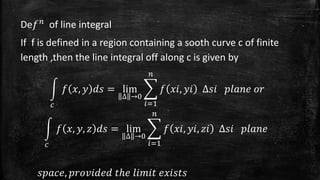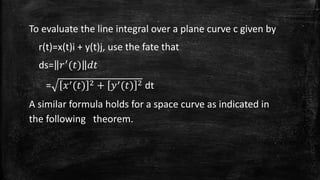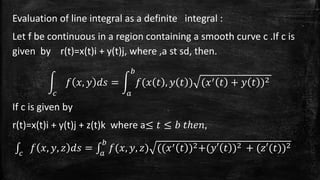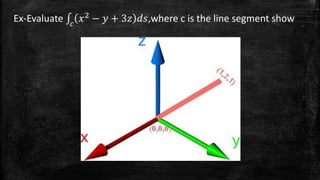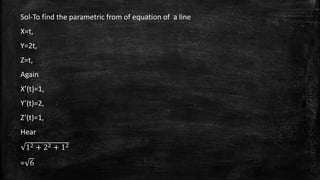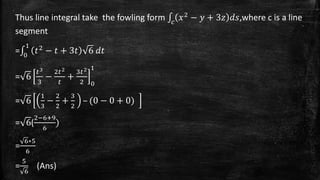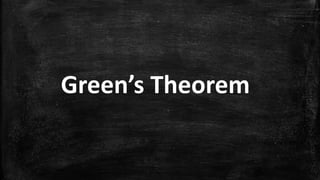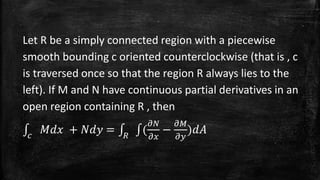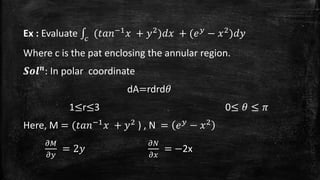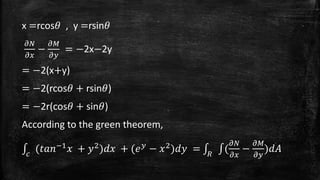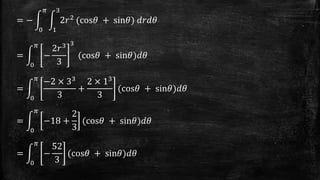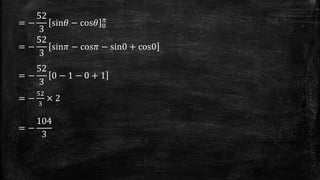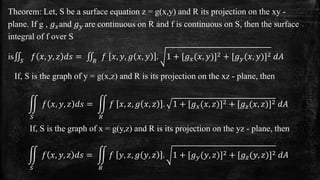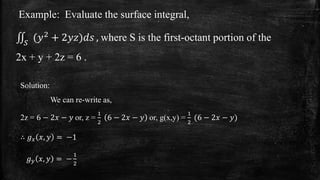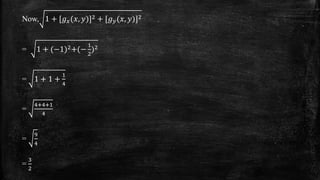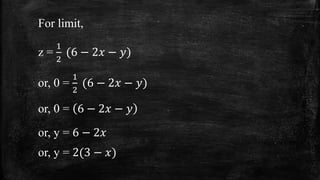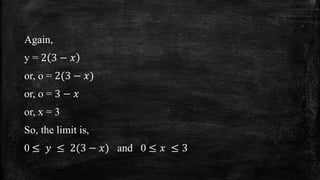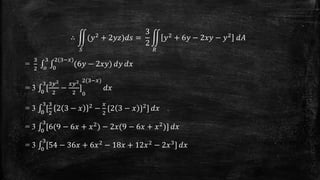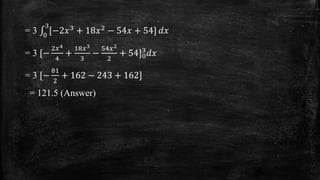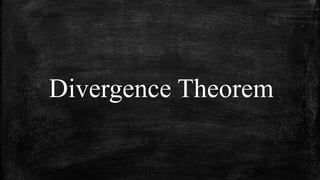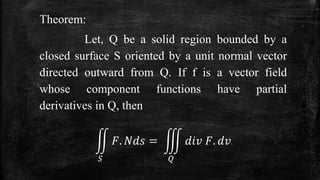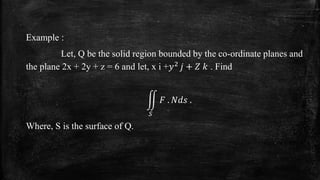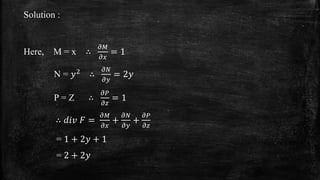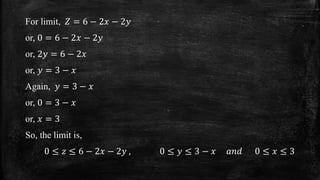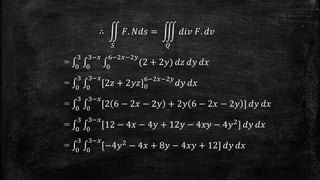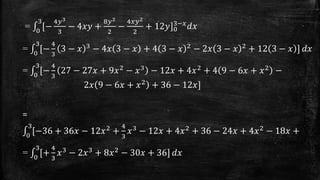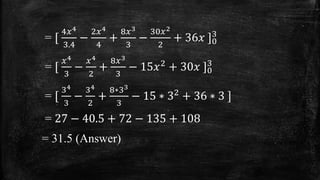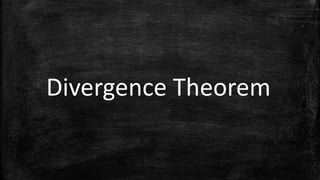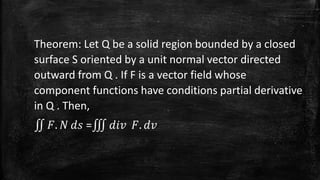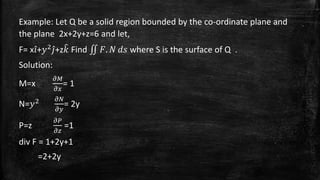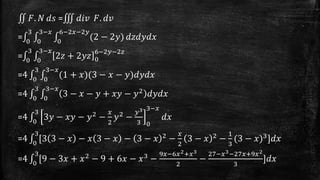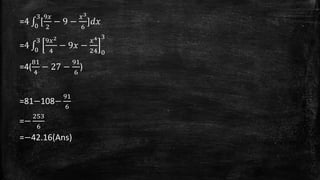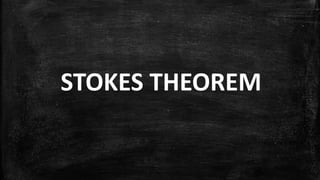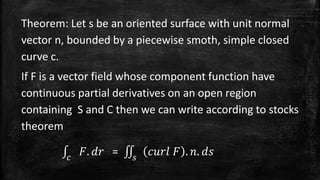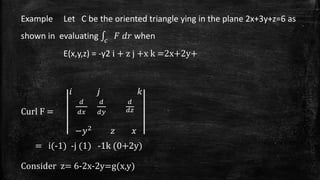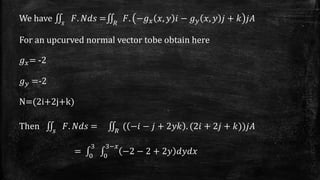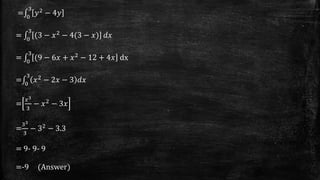1 von 98

### Presentation on calculus

• 1. Welcome to Our Mathematics Presentation
• 3. Group Members Name ID Name ID Md. Mouuin Uddin 12206003 Md. Raziur Rahman 12206026 Md. Shakkik Zunaed 12206004 Faysal Ahmad 12206030 Kazi Nourjahan Nipun 12206005 Md. Alamgir Hossain 12206035 Abdullah Al Naser 12206006 Abu Hossain Basri 12206036 Md.Rasheduzzaman 12206007 Manik Chandra Roy 12206039 Md. Hassan Shahriar 12206013 Md. Shariful Haque Robin 12206049 Md. Ashraful Islam 12206015 Md. Saifullah 12206051 Hossain Mohammad Jakaria 12206018 Md. Mahmuddujjaman 12206062 Imrana Khaton Eva 12206019 Arun Chandra Acharjee 12206066 Md. Saddam Hussein 12206023 Md. Oliullah Sheik 12206067 Joyantika Saha 12206025 Md. Rabiul Hasan 12206069 Rajesh Chandra Barman 12206071
• 4. Topic Topic 1. Distance Between Points, Plane and Line 9. Tangent Plane and Normal Line 2. Distance Between Two Parallel Planes 10. Curl of a vector field 3. Distance Between a Point a Line in Space 11. Curl and Divergence 4. Vector Value Function 12. Line Integral 5. Velocity and Acceleration 13. GreenтАЩs Theorem 6. Tangent vector 14. Surface Integral 7. Arc Length and Curvature 15. Divergence Theorem 8. The Gradient of a Function of Two Variables 16. Stokes Theorem
• 5. Distance Between Points Plane and Line
• 6. Theorem: The distance between a plane and point Q (Not in plane) is, D= эСГэСЯэСЬэСЧ эСГэСД = эСГэСД.эСЫ эСЫ , where P is a point in the plane and n is the normal in the plane. To find a point in the plane given by, ax+by+cz+d=0 (aтЙа0), let y=0 and z=0, then from the equation, ax+d=0, we can conclude that the point ( - эСС эСО , 0,0 ) lies in the plane and n is the normal to the plane.
• 7. Ex: Find the distance the point Q=( 1, 5, -5 ) and the plane given by 3xтАУy+2z=6. soln: We know that, эСЫ=< 3, -1, 2> is normal to the give plane. To find a point in the plane, let y=0 , z=0 and obtain the point P=( 2, 0, 0) the vector, эСГэСД=< 1-2, 5-0, -4-0 > =< -1, 5, -4 >
• 8. Using the distance formula produces, D= эСГэСД .эСЫ эСЫ = < тИТ1, 5,тИТ4> .< 3,тИТ1, 2> 32+(тИТ1)2+22 = тИТ1 тИТ5 тИТ8 9+1+4 тИТ16 = 14 = 16 14 =4.276 (Answer)
• 9. Ex: Find the distance the point Q=(1, 2, 3) and plane given by 2x-y+z=4. Soln: We know that эСЫ=<2, -1, 1> is normal to the given plane. To find a point in the plane, let y=0, z=0 and obtain the point P=(2, 0, 0). The vector, эСГэСД=<1-2, 2-0, 3-0> =<-1, 2, 3>
• 10. Using the distance formula produce D= эСГэСД . эСЫ эСЫ = <тИТ1, 2, 3> .<2,тИТ1, 1> 22+(тИТ1)2+12 тИТ2тИТ2+3 = 4+1+1 = тИТ1 6 = 1 6 =0.408 (Answer)
• 11. Distance Between Two Parallel Planes
• 12. Theorem: We can determine that the distance between the point Q = (x0, y0, z0) and the plane given by ax+by+cz+d=0 is эСОэСе0+эСПэСж0+эСРэСз0+эСС D = эСО2+эСП2+эСР2 Distance between point and plane where P= (x,y,z) is a point on the plane and d= - (ax1 + by1 + cz1) or ax1 + by1 + cz1 +d = 0
• 13. Ex: Find the distance between the two parallel planes given by 3x тАУ y + 2z тАУ 6 = 0 and 6x тАУ 2y + 4z + 4= 0 Soln: Let, y=0, z=0 Q=(2,0,0) And here we given, a=6, b= - 2, c= 4, d = 4
• 14. Distance between two parallel plane is эСОэСе0+эСПэСжD= 0+эСРэСз0 эСО2+эСП2+эСР2 D= 6тИЧ2 + тИТ2тИЧ0 + 4тИЧ0 +4 62+(тИТ2)2+42 12+4 = 36+4+16 = 16 56 = 16 56 =2.138 (Answer)
• 15. Ex: Find the distance between the two parallel planes given by x тАУ 3y + 4z =10 , x тАУ 3y + 4z -6 =0. Soln: Let, y=0 and z=0 Q=(10,0,0) And here we given X= 1, y= -3, z= 4, d= -6
• 16. Distance between two parallel plane эСОэСе0+эСПэСжD= 0+эСРэСз0 эСО2+эСП2+эСР2 D= 0тИЧ1 + тИТ3тИЧ0 + 4тИЧ0 +(тИТ6) 12+(тИТ3)2+42 10тИТ6 = 1+9+16 = 4 26 = 4 26 =0.7845 (Answer)
• 17. Distance Between a Point a Line in Space
• 18. Theorem: The distance between a point Q and a эСГэСД├ЧэСИ line in space is given by D= эСИ ,where эСИ is the directional vector for line and P is a point on the line.
• 19. Ex : Find the distance between the point P= (3,тИТ1,4) and the line given by x =тИТ2+3t , y = тИТ2t, z = 1+4t. эСЖэСЬэСЩэСЫ: Using the direction number 3,тИТ2, 4 We know that the direction vector for the line is эСИ=< 3, тИТ2, 4 > To find a point on the line , let t= 0 and we obtain P = (тИТ2 , 0, 1) point on the line. Then эСГэСД=< 3тИТ (тИТ2) , тИТ1тИТ0 , 4тИТ1 > =< 5, тИТ1 ,3 > and we can form
• 20. эСГэСД ├Ч эСИ = эСЦ эСЧ эСШ 5 тИТ1 3 3 тИТ2 4 = эСЦ ( тИТ4 + 6) тИТ эСЧ (20тИТ9) +эСШ (тИТ10 + 3) = 2 эСЦ тИТ 11эСЧ тИТ 7эСШ =< 2, тИТ11, тИТ7 > D = эСГэСД├ЧэСИ эСИ = 22+(тИТ11)2+(тИТ7)2 9+4+16 = 4+121+49 29 = 174 29 =2.45 (Answer)
• 21. Vector Value Function
• 22. A function of the form r(t)= f(t) эСЦ +g(t) эСЧ and r(t)= f(t) эСЦ +g(t) эСЧ + h(t)эСШ is a vector valued function, where the component of f, g, h are real valued function of the parameter t, r(t)= эСУ эСб , эСФ(эСб) or r(t)= эСУ эСб , эСФ эСб , тДО(эСб)
• 23. Differention of a vector valued function: Defn: The derivative of a vector valued function r is defined by r┬┤(t)= lim ╬ФэСбтЖТ0 эСЯ эСб+╬ФэСб тИТэСЯ(эСб) ╬ФэСб for all t for which the limit exists. If r┬┤(c) exists for all c in open interval I, then r is differentiable on the interval I. Differentiability of vector valued intervals by considering one side limits.
• 24. Ex: Find the derivative of each of the following vector-valued function (i) r(t)= t2 эСЦ 4 эСЧ Soln: эССэСЯ / r┬┤(t)=2t эСЦ эССэСб (ii) r(t)= 1 эСб эСЦ + lnt эСЧ+тДо2t эСШ Soln: эССэСЯ = эССэСб 1 эСб2 эСЦ + 1 эСб эСЧ+ 2тДо2t эСШ
• 25. Velocity and Acceleration
• 26. Defn: If x and y are twice differentiable functions of t and r is a vector-valued function given by r(t)= x(t) эСЦ + y(t) эСЧ, then the velocity vector , acceleration vector , and speed at time t are follows Velocity = эСг эСб = r┬┤(t)= x┬┤(t) эСЦ + y┬┤(t) эСЧ Acceleration n= a(t)= r┬┤┬┤(t)= x┬┤┬┤(t) эСЦ + y┬┤┬┤(t) эСЧ Speed = эСг эСб = r┬┤(t) = [x┬┤(t)]2+[y┬┤(t)]2
• 27. Example: Find the velocity and acceleration vector when t=0 and t=3 for the vector valued function r(t)= <эСТэСб эСРэСЬэСаэСб, эСТэСбэСаэСЦэСЫэСб, эСТэСб >. r(t) = эСТэСб эСРэСЬэСаэСб эСЦ + эСТэСбэСаэСЦэСЫэСб эСЧ + эСТэСб эСШ Velocity = эСТэСб эСС эССэСе эСРэСЬэСаэСб + эСРэСЬэСаэСб эСС эССэСе эСТэСб эСЦ + эСТэСб эСШ = (тИТэСТэСбэСаэСЦэСЫэСб + эСТэСб эСРэСЬэСаэСб ) эСЦ +(эСТэСб эСРэСЬэСаэСб + эСТэСбэСаэСЦэСЫэСб) эСЧ + эСТэСб эСШ = < эСТэСб эСРэСЬэСаэСб тИТ эСаэСЦэСЫэСб , эСТэСб (эСаэСЦэСЫэСб + эСРэСЬэСаэСб) , эСТэСб > (Answer)
• 28. Speed = r╦К(t) = эСТэСб эСРэСЬэСаэСб тИТ эСТэСб эСаэСЦэСЫэСб 2 + (эСТэСбэСаэСЦэСЫэСб + эСТэСбэСРэСЬэСаэСб)2+(эСТэСб)2 = эСТэСб 2 эСРэСЬэСаэСб тИТ эСаэСЦэСЫэСб 2 + эСТэСб 2 эСаэСЦэСЫэСб + эСРэСЬэСаэСб 2 + эСТэСб 2 = эСТэСб 1 тИТ 2эСаэСЦэСЫэСб эСРэСЬэСаэСб + эСО + 2эСаэСЦэСЫэСб эСРэСЬэСаэСб + 1 = эСТэСб 3 (Answer)
• 29. Tangent vector
• 30. Defn : Let C be a smooth curve represented by r on an open interval I . The unit tangent vector T(t) at t is Defined to be T(t)= эСЯ╠Б(эСб) эСЯ╠Б(эСб) , эСЯ╠Б(эСб) тЙа 0
• 31. Ex: Find the unit tangent vector to the curve given by r(t)=t эСЦ +эСб2 эСЧ when t=1 Slon : Given r(t)=t эСЦ +эСб2 эСЧ эСЯ╠Б(эСб)= эСЦ + 2t эСЧ эСЯ╠Б(эСб) = 12+(2эСб)2 = 1 + 4эСб2
• 32. The tangen vector is T(t)= эСЯ╠Б(эСб) эСЯ╠Б(эСб) = 1 1+4эСб2 (эСЦ + 2t эСЧ ) The unit tangent vector at t=1 is T(1) = 1 5 (эСЦ + 2 эСЧ ) (Answer)
• 33. Arc Length and Curvature
• 34. Definition of Arc Length: The arc length of a smooth plane curve c by the parametric equations x=x(t) and y=(t), a тЙд t тЙд b is эСП S= эСО [эСетА▓(эСб)]2+[эСжтА▓(эСб)]2 dt . Where c is given by r(t)= x(t)├о + y(t)─╡ . We can rewrite this equation for Arc эСП Length as, s= эСЯтА▓ эСб эССэСб эСО
• 35. Ex: Find the arc length of the curve given by r(t)=cost ├о + sint ─╡ from t=0 to t=2╧А Solution: Here, x(t)=cost y(t)= sint XтАЩ(t)=-sint yтАЩ(t)=cost We have эСП S= эСО [эСетА▓(эСб)]2+[эСжтА▓(эСб)]2 dt
• 36. 2эЬЛ S= 0 эСаэСЦэСЫ2эСб + эСРэСЬэСа2эСб dt 2эЬЛ S= 0 1dt 2эЬЛ S= 0 1dt S=[эСб]0 2 эЬЛ S=2╧А (Answer)
• 37. Curvature: let c be a smooth curve (in the plane or in space) given by r(s), where s is the arc length parameter. The curvature at s is given by эССэСЗ эССэСа K=|| || K=||TтАЩ(s)||
• 38. Ex: Find the curvature of the line given by r(s)= (3- 3 5 s) ├о + 4 5 s ─╡ Solution: 3 5 r(s) = (3- s) ├о + 4 5 s ─╡ rтАЩ(s) = ( 0- 3 5 )├о + 4 5 ─╡ ||rтАЩ(s)|| = (тИТ 3 5 )2+( 4 5 )2 = 9 25 + 16 25
• 39. = 25 25 =1 T(s) = эСЯтА▓(эСа) ||эСЯтА▓(эСа)|| = тИТ 3 5 ├о + 4 5 ─╡ TтАЩ(s) = 0.├о+0.─╡ ||TтАЩ(S)|| = 0 K = ||TтАЩ(S)|| = 0 (Answer)
• 40. The Gradient of a Function of Two Variables
• 41. Definition : Let Z=f(x,y) be a function of x and y such that эСУэСе and эСУэСж exit. Then the gradient of f, denoted by эЫ╗эСУ( эСе, эСж ) ,is the vector of эЫ╗эСУ эСе, эСж = эСУэСе( x,y )эСЦ + эСУэСж ( x,y) эСЧ We read эЫ╗эСУ эСОэСа тА▓тА▓эССэСТэСЩэСУтА▓тА▓. Another notation for the gradient is grad эСУ эСе, эСж .
• 42. Ex: Find the gradient of эСУ эСе, эСж = эСж эСЩэСЫ эСе + эСеэСж2 at the point (1,2). эС║эТРэТНэТП: Grad f or эЫ╗эСУ = эСУэСе ( x,y )эСЦ + эСУэСж ( x,y) эСЧ тАжтАжтАжтАжтАжтАжтАжтАжтАжтАж(i) Now, эСУэСе= эСж эСе + эСж2 , эСУэСж = эСЩэСЫ эСе + 2эСеэСж тИ┤ эСУэСе( 1,2)= 2 + 22 = 6
• 43. тИ┤ эСУэСж ( 1,2) = ln 1 + 2 тИЩ 1 тИЩ 2 = 4 From (i) we get, эЫ╗эСУ = 6эСЦ + 4эСЧ (Ans:)
• 44. Tangent Plane and Normal Line
• 45. Definition: Let F be differentiable at the point P= (эСе0 ,эСж0 ,эСз0 ) u the surface s given by F(x, y, z) = 0 such that эЫ╗эР╣ эСе0 ,эСж0 ,эСз0 тЙа 0. 1). The plane through P that is normal to эЫ╗эР╣ эСе0 ,эСж0 ,эСз0 is called the tangent plane to S at P. 2). The line through P having the direction of эЫ╗эР╣ эСе0 ,эСж0 ,эСз0 is called the normal line to S at P. If F is differentiable at эСе0 ,эСж0 ,эСз0 then an equation of the tangent plane to the surface given by эР╣ эСе, эСж, эСз = 0 at эСе0 ,эСж0 ,эСз0 is эР╣эСе эСе0 ,эСж0 ,эСз0 (xтИТэСе0)+ эР╣эСж эСе0 ,эСж0 ,эСз0 (yтИТэСж0) +эР╣эСз эСе0 ,эСж0 ,эСз0 (zтИТэСз0)= 0.
• 46. Ex : Find an equation of the tangent plane to the hyperboloid given by at эСз2тИТ2эСе2 тИТ 2эСж2 = 12 the point (1,тИТ1, 4) . эС║эТРэТНэТП: An the equation of the tangent plane to the Given hyperboloid can be rewrite as эР╣ эСе, эСж, эСз = 12 тИТ эСз2 +2эСе2 + 2эСж2
• 47. эР╣эСе эСе, эСж, эСз =4x тИ┤ эР╣эСе 1, тИТ1, 4 =4 эР╣эСж эСе, эСж, эСз =4y тИ┤ эР╣эСж 1, тИТ1, 4 = тИТ4 эР╣эСз эСе, эСж, эСз = тИТ2эСз тИ┤ эР╣эСз 1, тИТ1, 4 = тИТ8 The tangent plane is , 4(xтИТ1) + тИТ4 (y+1) + ( тИТ8)(zтИТ4) = 0 тЗТ4xтИТ4тИТ4yтИТ4тИТ8z+32 = 0 тЗТ4xтИТ4yтИТ8z+24 = 0 (Answer)
• 48. Curl of a vector field
• 49. Curl of a vector field: The curl of the F(x,y,z)= MэСЦ +NэСЧ +pэСШ is Curl F(x,y,z)= эЫ╗ x F(x,y,z) = ( эЬХэСЭ эЬХэСж - эЬХэСБ эЬХэСз ) эСЦ -( эЬХэСЭ эЬХэСе - эЬХэСА эЬХэСз ) эСЧ +( эЬХэСБ эЬХэСе - эЬХэСГ эЬХэСж ) эСШ If curl F=0, then F is irrational. эЫ╗ x F(x,y,z)= i эСЧ эСШ эЬХ эЬХ эЬХэСе эЬХэСж эЬХ эЬХэСз эСА эСБ эСГ
• 50. Example: Show that the vector field F is irrotational where F(x,y,z)= 2xyi+ (x2+z2)j + 2zyk Given, F(x,y,z)= 2xyi+ (x2+z2)j + 2zyk эЫ╗ x F(x,y,z)= i эСЧ эСШ эЬХ эЬХ эЬХэСе эЬХэСж эЬХ эЬХэСз 2xy (x2 + z2) 2zy = i{ эЬХ эЬХэСж эЬХ эЬХэСз . 2zy- (x2+z2)} тАУ j( эЬХ эЬХэСе .2zy - эЬХ эЬХэСз .2xy) +k{ эЬХ эЬХэСе эЬХ эЬХэСж (x2+z2) - .2xy} =i(2z-2z) тАУj(0-0) +k(2x-2x) =0i + 0j + 0k =0 If curl F=0, then the vector field F is irrational.
• 51. Curl and Divergence
• 52. Curl; Curl эР╣ = эЫ╗ ├Ч эР╣ эСе, эСж, эСз = эЬХ эЬХэСе + эЬХ эЬХэСж + эЬХ эЬХэСз ├Ч эСЦ + эСЧ + эСШ If эСРэСвэСЯэСЩ эР╣ = 0 эСЗтДОэСТэСЫ эР╣ is irrational.
• 53. Problem: Find the curl F of the followings F(x, y, z) = эСТтИТэСеэСжэСз(эСЦ + эСЧ + эСШ ) and point (3,2,0) Solution: F(x, y, z) = эСТтИТэСеэСжэСз(эСЦ + эСЧ + эСШ ) F x, y, z = эСТтИТэСеэСжэСзэСЦ + эСТтИТэСеэСжэСзэСЧ + эСТтИТэСеэСжэСзэСШ
• 54. эСРэСвэСЯэСЩ эР╣(эСе, эСж, эСз) = эЫ╗ ├Ч эР╣(эСе, эСж, эСз) = эСЦ эСЧ эСШ эЬХ эЬХ эЬХэСе эЬХэСж эЬХ эЬХэСз эСТтИТэСеэСжэСз эСТтИТэСеэСжэСз эСТтИТэСеэСжэСз = (тИТэСеэСзэСТтИТэСеэСжэСз + эСеэСжэСТтИТэСеэСжэСз)эСЦ тАУ тИТэСжэСзэСТтИТэСеэСжэСз + эСеэСжэСТтИТэСеэСжэСз эСЧ + (тИТэСжэСзэСТтИТэСеэСжэСз + эСеэСзэСТтИТэСеэСжэСз) эСШ At point (3, 2, 0) Curl F= (0+6эСТ0)эСЦ тИТ 0 + 6эСТ0 эСЧ + (0 + 0)эСШ =6эСЦ + 6эСЧ (Ans)
• 55. Divergence: div F = эЫ╗. эР╣(эСе, эСж, эСз) div F= эЬХэСА эЬХэСе + эЬХэСБ эЬХэСж + эЬХэСГ эЬХэСз where F(x,y,z) = MэСЦ + эСБэСЧ + эСГэСШ If div F=0, then it is said to be divergence free. Relation between curl & divergence is div(curlF)=0.
• 56. Problem: Find the divergence of эР╣ эСе, эСж, эСз = эСеэСжэСз эСЦ тИТ эСжэСЧ + эСзэСШ and also find the relation between curl & divergence. Solution: Given that эР╣ эСе, эСж, эСз = эСеэСжэСз эСЦ тИТ эСжэСЧ + эСзэСШ эСРэСвэСЯэСЩ эР╣ эСе, эСж, эСз = эЫ╗ ├Ч эР╣ эСе, эСж, эСз = эСЦ эСЧ эСШ эЬХ эЬХ эЬХэСе эЬХэСж эЬХ эЬХэСз эСеэСжэСз тИТэСж эСз
• 57. = (0-0)эСЦ тИТ 0 тИТ эСеэСж эСЧ + (0 тИТ эСеэСз)эСШ Curl F(эСе, эСж, эСз) = эСеэСжэСЧ тИТ эСеэСзэСШ We know that div F= эЬХэСЪ эЬХэСе + эЬХэСЫ эЬХэСж + эЬХэСЭ эЬХэСз Here, m=xyz n= -y p= z эЬХэСЪ эЬХэСе = эСжэСз эЬХэСЫ эЬХэСе = тИТ1 эЬХэСЭ эЬХэСе = 1 эССэСЦэСгэР╣ эСе, эСж, эСз = эСжэСз тИТ 1 + 1 = эСжэСз At the point (1,2,1) эССэСЦэСг эР╣ эСе, эСж, эСз = 2 ├Ч 1 = 2 (Ans)
• 58. Now div (curl F) = эЬХэСЪ эЬХэСе + эЬХэСЫ эЬХэСж + эЬХэСЭ эЬХэСз Here, эСЪ = 0 эСЫ = эСеэСж эСЭ = тИТэСеэСз эЬХэСЪ эЬХэСе = 0 эЬХэСЫ эЬХэСж = эСе эЬХэСЭ эЬХэСз = тИТэСе Relation between curl & divergence div (curl F)=0 + эСе тИТ эСе = 0
• 59. Line Integral
• 60. DeэСУэСЫ of line integral If f is defined in a region containing a sooth curve c of finite length ,then the line integral off along c is given by эСР эСУ эСе, эСж эССэСа = lim ╬Ф тЖТ0 эСЫ эСЦ=1 эСУ эСеэСЦ, эСжэСЦ ╬ФэСаэСЦ эСЭэСЩэСОэСЫэСТ эСЬэСЯ эСР эСУ эСе, эСж, эСз эССэСа = lim ╬Ф тЖТ0 эСЫ эСЦ=1 эСУ эСеэСЦ, эСжэСЦ, эСзэСЦ ╬ФэСаэСЦ эСЭэСЩэСОэСЫэСТ эСаэСЭэСОэСРэСТ, эСЭэСЯэСЬэСгэСЦэССэСТэСС эСбтДОэСТ эСЩэСЦэСЪэСЦэСб эСТэСеэСЦэСаэСбэСа
• 61. To evaluate the line integral over a plane curve c given by r(t)=x(t)i + y(t)j, use the fate that ds= эСЯтА▓(эСб) эССэСб = эСетА▓(эСб) 2 + эСжтА▓(эСб) 2 dt A similar formula holds for a space curve as indicated in the following theorem.
• 62. Evaluation of line integral as a definite integral : Let f be continuous in a region containing a smooth curve c .If c is given by r(t)=x(t)i + y(t)j, where ,a st sd, then. эСР эСУ эСе, эСж эССэСа = эСП эСУ(эСе эСб , эСж эСб ) (эСетА▓ эСб + эСж эСб )2 эСО If c is given by r(t)=x(t)i + y(t)j + z(t)k where aтЙд эСб тЙд эСП эСбтДОэСТэСЫ, эСП эСУ(эСе, эСж, эСз) ((эСетА▓ эСб )2+(эСжтА▓ эСб )2 + (эСзтА▓(эСб))2 эСР эСУ эСе, эСж, эСз эССэСа = эСО
• 63. Ex-Evaluate эСР эСе2 тИТ эСж + 3эСз эССэСа,where c is the line segment show
• 64. Sol-To find the parametric from of equation of a line X=t, Y=2t, Z=t, Again XтАЩ(t)=1, YтАЩ(t)=2, ZтАЩ(t)=1, Hear 12 + 22 + 12 = 6
• 65. Thus line integral take the fowling form эСР эСе2 тИТ эСж + 3эСз эССэСа,where c is a line segment 1 = 0 эСб2 тИТ эСб + 3эСб 6 эССэСб = 6 эСб3 3 тИТ 2эСб2 эСб + 1 3эСб2 2 0 = 6 1 3 тИТ 2 2 + 3 2 тАУ (0 тИТ 0 + 0) = 6( 2тИТ6+9 6 ) 6тИЧ5 = 6 = 5 6 (Ans)
• 66. GreenтАЩs Theorem
• 67. Let R be a simply connected region with a piecewise smooth bounding c oriented counterclockwise (that is , c is traversed once so that the region R always lies to the left). If M and N have continuous partial derivatives in an open region containing R , then эСР эСАэССэСе + эСБэССэСж = эСЕ ( эЬХэСБ эЬХэСе тИТ эЬХэСА эЬХэСж )эССэР┤
• 68. Ex : Evaluate эСР (эСбэСОэСЫтИТ1эСе + эСж2)эССэСе + (эСТэСж тИТ эСе2)эССэСж Where c is the pat enclosing the annular region. эС║эТРэТНэТП: In polar coordinate dA=rdrdэЬГ 1тЙдrтЙд3 0тЙд эЬГ тЙд эЬЛ Here, M = (эСбэСОэСЫтИТ1эСе + эСж2 ) , N = эСТэСж тИТ эСе2 эЬХэСА эЬХэСж = 2эСж эЬХэСБ эЬХэСе = тИТ2x
• 69. x =rcosэЬГ , y =rsinэЬГ эЬХэСБ эЬХэСе тИТ эЬХэСА эЬХэСж = тИТ2xтИТ2y = тИТ2(x+y) = тИТ2(rcosэЬГ + rsinэЬГ) = тИТ2r(cosэЬГ + sinэЬГ) According to the green theorem, эСР (эСбэСОэСЫтИТ1эСе + эСж2)эССэСе + (эСТэСж тИТ эСе2)эССэСж = эСЕ ( эЬХэСБ эЬХэСе тИТ эЬХэСА эЬХэСж )эССэР┤
• 70. = тИТ эЬЛ 0 3 2эСЯ2 (cosэЬГ + sinэЬГ) эССэСЯэССэЬГ 1 = эЬЛ 0 тИТ 2эСЯ3 3 3 (cosэЬГ + sinэЬГ)эССэЬГ = эЬЛ тИТ2 ├Ч 33 0 3 + 2 ├Ч 13 3 (cosэЬГ + sinэЬГ)эССэЬГ = эЬЛ 0 тИТ18 + 2 3 (cosэЬГ + sinэЬГ)эССэЬГ = эЬЛ 0 тИТ 52 3 (cosэЬГ + sinэЬГ)эССэЬГ
• 71. = тИТ 52 3 эЬЛ sinэЬГ тИТ cosэЬГ 0 = тИТ 52 3 sinэЬЛ тИТ cosэЬЛ тИТ sin0 + cos0 = тИТ 52 3 0 тИТ 1 тИТ 0 + 1 = тИТ 52 3 ├Ч 2 = тИТ 104 3
• 72. Surface Integral
• 73. Theorem: Let, S be a surface equation z = g(x,y) and R its projection on the xy - plane. If g , эСФэСеand эСФэСж are continuous on R and f is continuous on S, then the surface integral of f over S is эСЖ эСУ эСе, эСж, эСз эССэСа = эСЕ эСУ эСе, эСж, эСФ эСе, эСж . 1 + [эСФэСе(эСе, эСж)]2 + [эСФэСж (эСе, эСж)]2 эССэР┤ If, S is the graph of y = g(x,z) and R is its projection on the xz - plane, then эСЖ эСУ эСе, эСж, эСз эССэСа = эСЕ эСУ эСе, эСз, эСФ эСе, эСз . 1 + [эСФэСе(эСе, эСз)]2 + [эСФэСз(эСе, эСз)]2 эССэР┤ If, S is the graph of x = g(y,z) and R is its projection on the yz - plane, then эСЖ эСУ эСе, эСж, эСз эССэСа = эСЕ эСУ эСж, эСз, эСФ эСж, эСз . 1 + [эСФэСж(эСж, эСз)]2 + [эСФэСз(эСж, эСз)]2 эССэР┤
• 74. Example: Evaluate the surface integral, эСЖ (эСж2 + 2эСжэСз)эССэСа , where S is the first-octant portion of the 2x + y + 2z = 6 . Solution: We can re-write as, 2z = 6 тИТ 2эСе тИТ эСж or, z = 1 2 6 тИТ 2эСе тИТ эСж or, g(x,y) = 1 2 (6 тИТ 2эСе тИТ эСж) тИ┤ эСФэСе эСе, эСж = тИТ1 эСФэСж эСе, эСж = тИТ 1 2
• 75. Now, 1 + [эСФэСе(эСе, эСж)]2 + [эСФэСж(эСе, эСж)]2 = 1 + (тИТ1)2+(тИТ 1 2 )2 = 1 + 1 + 1 4 = 4+4+1 4 = 9 4 = 3 2
• 76. For limit, z = 1 2 (6 тИТ 2эСе тИТ эСж) or, 0 = 1 2 (6 тИТ 2эСе тИТ эСж) or, 0 = 6 тИТ 2эСе тИТ эСж or, y = 6 тИТ 2эСе or, y = 2(3 тИТ эСе)
• 77. Again, y = 2 3 тИТ эСе or, o = 2(3 тИТ эСе) or, o = 3 тИТ эСе or, x = 3 So, the limit is, 0 тЙд эСж тЙд 2(3 тИТ эСе) and 0 тЙд эСе тЙд 3
• 78. тИ┤ эСЖ (эСж2 + 2эСжэСз)эССэСа = 3 2 эСЕ эСж2 + 6эСж тИТ 2эСеэСж тИТ эСж2 эССэР┤ = 3 2 3 эСЬ 2(3тИТэСе) 0 (6эСж тИТ 2эСеэСж) эССэСж эССэСе 3 [ = 3 0 3эСж2 2 тИТ эСеэСж2 2 2(3тИТэСе) ] 0 эССэСе 3 [ = 3 0 3 2 2 3 тИТ эСе 2 тИТ эСе 2 {2 3 тИТ эСе }2] эССэСе 3 [6(9 тИТ 6эСе + эСе2) тИТ 2эСе(9 тИТ 6эСе + эСе2)] эССэСе = 3 0 3 [54 тИТ 36эСе + 6эСе2 тИТ 18эСе + 12эСе2 тИТ 2эСе3] эССэСе = 3 0
• 79. 3 [тИТ2эСе3 + 18эСе2 тИТ 54эСе + 54] эССэСе = 3 0 = 3 [тИТ 2эСе4 4 + 18эСе3 3 тИТ 54эСе2 2 3эССэСе + 54]0 = 3 [тИТ 81 2 + 162 тИТ 243 + 162] = 121.5 (Answer)
• 80. Divergence Theorem
• 81. Theorem: Let, Q be a solid region bounded by a closed surface S oriented by a unit normal vector directed outward from Q. If f is a vector field whose component functions have partial derivatives in Q, then эСЖ эР╣. эСБэССэСа = эСД эССэСЦэСг эР╣. эССэСг
• 82. Example : Let, Q be the solid region bounded by the co-ordinate planes and the plane 2x + 2y + z = 6 and let, x i +эСж2 эСЧ + эСН эСШ . Find эСЖ эР╣ . эСБэССэСа . Where, S is the surface of Q.
• 83. Solution : Here, M = x тИ┤ эЬХэСА эЬХэСе = 1 N = эСж2 тИ┤ эЬХэСБ эЬХэСж = 2эСж P = Z тИ┤ эЬХэСГ эЬХэСз = 1 тИ┤ эССэСЦэСг эР╣ = эЬХэСА эЬХэСе + эЬХэСБ эЬХэСж + эЬХэСГ эЬХэСз = 1 + 2эСж + 1 = 2 + 2эСж
• 84. For limit, эСН = 6 тИТ 2эСе тИТ 2эСж or, 0 = 6 тИТ 2эСе тИТ 2эСж or, 2эСж = 6 тИТ 2эСе or, эСж = 3 тИТ эСе Again, эСж = 3 тИТ эСе or, 0 = 3 тИТ эСе or, эСе = 3 So, the limit is, 0 тЙд эСз тЙд 6 тИТ 2эСе тИТ 2эСж , 0 тЙд эСж тЙд 3 тИТ эСе эСОэСЫэСС 0 тЙд эСе тЙд 3
• 85. тИ┤ эСЖ эР╣. эСБэССэСа = эСД эССэСЦэСг эР╣. эССэСг 3 = 0 3тИТэСе 0 6тИТ2эСетИТ2эСж 0 (2 + 2эСж) эССэСз эССэСж эССэСе 3 = 0 3тИТэСе 0 6тИТ2эСетИТ2эСжэССэСж эССэСе [2эСз + 2эСжэСз]0 3 = 0 3тИТэСе 0 [2 6 тИТ 2эСе тИТ 2эСж + 2эСж 6 тИТ 2эСе тИТ 2эСж ] эССэСж эССэСе 3 = 0 3тИТэСе 0 [12 тИТ 4эСе тИТ 4эСж + 12эСж тИТ 4эСеэСж тИТ 4эСж2] эССэСж эССэСе 3 = 0 3тИТэСе 0 [тИТ4эСж2 тИТ 4эСе + 8эСж тИТ 4эСеэСж + 12] эССэСж эССэСе
• 86. 3 [тИТ = 0 4эСж3 3 тИТ 4эСеэСж + 8эСж2 2 тИТ 4эСеэСж2 2 + 12эСж]03тИТэСеэССэСе 3 [тИТ = 0 4 3 3 тИТ эСе 3 тИТ 4эСе 3 тИТ эСе + 4 3 тИТ эСе 2 тИТ 2эСе 3 тИТ эСе 2 + 12 3 тИТ эСе ] эССэСе 3 [тИТ = 0 4 3 27 тИТ 27эСе + 9эСе2 тИТ эСе3 тИТ 12эСе + 4эСе2 + 4 9 тИТ 6эСе + эСе2 тИТ 2эСе 9 тИТ 6эСе + эСе2 + 36 тИТ 12эСе] = 3 [тИТ36 + 36эСе тИТ 12эСе2 + 0 4 3 эСе3 тИТ 12эСе + 4эСе2 + 36 тИТ 24эСе + 4эСе2 тИТ 18эСе + 3 [+ = 0 4 3 эСе3 тИТ 2эСе3 + 8эСе2 тИТ 30эСе + 36] эССэСе
• 87. = [ 4эСе4 3.4 тИТ 2эСе4 4 + 8эСе3 3 тИТ 30эСе2 2 + 36эСе ]0 3 = [ эСе4 3 тИТ эСе4 2 + 8эСе3 3 тИТ 15эСе2 + 30эСе ]0 3 = [ 34 3 тИТ 34 2 + 8тИЧ33 3 тИТ 15 тИЧ 32 + 36 тИЧ 3 ] = 27 тИТ 40.5 + 72 тИТ 135 + 108 = 31.5 (Answer)
• 88. Divergence Theorem
• 89. Theorem: Let Q be a solid region bounded by a closed surface S oriented by a unit normal vector directed outward from Q . If F is a vector field whose component functions have conditions partial derivative in Q . Then, эР╣. эСБ эССэСа = эССэСЦэСг эР╣. эССэСг
• 90. Example: Let Q be a solid region bounded by the co-ordinate plane and the plane 2x+2y+z=6 and let, F= xэСЦ +эСж2эСЧ +zэСШ Find эР╣.эСБ эССэСа where S is the surface of Q . Solution: M=x эЬХэСА эЬХэСе = 1 N=эСж2 эЬХэСБ эЬХэСж = 2y P=z эЬХэСГ эЬХэСз =1 div F = 1+2y+1 =2+2y
• 91. эР╣. эСБ эССэСа = эССэСЦэСг эР╣. эССэСг 3 = 0 3тИТэСе 0 6тИТ2эСетИТ2эСж 0 (2 тИТ 2эСж) эССэСзэССэСжэССэСе 3 = 0 3тИТэСе 0 6тИТ2эСжтИТ2эСз 2эСз + 2эСжэСз 0 3 =4 0 3тИТэСе 0 (1 + эСе)(3 тИТ эСе тИТ эСж)эССэСжэССэСе 3 =4 0 3тИТэСе 0 3 тИТ эСе тИТ эСж + эСеэСж тИТ эСж2 эССэСжэССэСе 3 3эСж тИТ эСеэСж тИТ эСж2 тИТ =4 0 эСе 2 эСж2 тИТ 3тИТэСе эСж3 3 0 эССэСе 3 [3 3 тИТ эСе тИТ эСе 3 тИТ эСе тИТ 3 тИТ эСе 2 тИТ =4 0 эСе 2 3 тИТ эСе 2 тИТ 1 3 (3 тИТ эСе)3]эССэСе 3 [9 тИТ 3эСе + эСе2 тИТ 9 + 6эСе тИТ эСе3 тИТ =4 0 9эСетИТ6эСе2+эСе3 2 тИТ 27тИТэСе3тИТ27эСе+9эСе2 3 ]эССэСе
• 92. 3 [ =4 0 9эСе 2 тИТ 9 тИТ эСе3 6 ]эССэСе 3 9эСе2 =4 0 4 тИТ 9эСе тИТ 3 эСе4 24 0 =4( 81 4 тИТ 27 тИТ 91 6 ) =81тИТ108тИТ 91 6 =тИТ 253 6 =тИТ42.16(Ans)
• 93. STOKES THEOREM
• 94. Theorem: Let s be an oriented surface with unit normal vector n, bounded by a piecewise smoth, simple closed curve c. If F is a vector field whose component function have continuous partial derivatives on an open region containing S and C then we can write according to stocks theorem эСР эР╣. эССэСЯ = эСа эСРэСвэСЯэСЩ эР╣ . эСЫ. эССэСа
• 95. Example Let C be the oriented triangle ying in the plane 2x+3y+z=6 as shown in evaluating эСР эР╣ эССэСЯ when E(x,y,z) = -y2 i + z j +x k =2x+2y+ Curl F = эСЦ эСЧ эСШ эСС эССэСе эСС эССэСж эСС эССэСз тИТэСж2 эСз эСе = i(-1) -j (1) -1k (0+2y) Consider z= 6-2x-2y=g(x,y)
• 96. We have эСа эР╣. эСБэССэСа = эСЕ эР╣. тИТэСФэСе эСе, эСж эСЦ тИТ эСФэСж эСе, эСж эСЧ + эСШ эСЧэР┤ For an upcurved normal vector tobe obtain here эСФэСе= -2 эСФэСж =-2 N=(2i+2j+k) Then эСа эР╣. эСБэССэСа = эСЕ ( тИТэСЦ тИТ эСЧ + 2эСжэСШ . (2эСЦ + 2эСЧ + эСШ))эСЧэР┤ 3 = 0 3тИТэСе 0 тИТ2 тИТ 2 + 2эСж эССэСжэССэСе
• 97. 3 эСж2 тИТ 4эСж = 0 3 (3 тИТ эСе2 тИТ 4(3 тИТ эСе) эССэСе = 0 3 (9 тИТ 6эСе + эСе2 тИТ 12 + 4эСе dx = 0 3 эСе2 тИТ 2эСе тИТ 3 эССэСе = 0 = эСе3 3 тИТ эСе2 тИТ 3эСе 33 3 = тИТ 32 тИТ 3.3 = 9- 9- 9 =-9 (Answer)